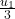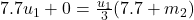## A 7.7 kg sphere makes a perfectly inelastic collision with a second sphere initially at rest. The composite system moves with a speed equal

Question

A 7.7 kg sphere makes a perfectly inelastic collision with a second sphere initially at rest. The composite system moves with a speed equal to one third the original speed of the 7.7 kg sphere. What is the mass of the second sphere

in progress 0
2 months 2021-07-31T08:23:00+00:00 2 Answers 1 views 0

The mass of the second sphere is 15.4 kg

Explanation:

Given;

mass of the first sphere, m₁ = 7.7 kg

initial velocity of the second sphere, u₂ = 0

let mass of the second sphere =  m₂

let the initial velocity of the first sphere = u₁

final velocity of the composite system, v = ¹/₃ x u₁ =From the principle of conservation of linear momentum;

Total momentum before collision = Total momentum after collision

m₁u₁ + m₂u₂ = v(m₁ + m₂)

Substitute the given values;Divide through by u₁

7.7 = ¹/₃(7.7 + m₂)

multiply both sides by 3

23.1 = 7.7 + m₂

m₂ = 23.1 – 7.7

m₂ = 15.4 kg

Therefore, the mass of the second sphere is 15.4 kg

15.4 kg.

Explanation:

From the law of conservation of momentum,

Total momentum before collision = Total momentum after collision

mu+m’u’ = V(m+m’)……………….. Equation 1

Where m = mass of the first sphere, m’ = mass of the second sphere, u = initial velocity of the first sphere, u’ = initial velocity of the second sphere, V = common velocity of both sphere.

Given: m = 7.7 kg, u’ = 0 m/s (at rest)

Let: u = x m/s, and V = 1/3x m/s

Substitute into equation 1

7.7(x)+m'(0) = 1/3x(7.7+m’)

7.7x = 1/3x(7.7+m’)

7.7 = 1/3(7.7+m’)

23.1 = 7.7+m’

m’ = 23.1-7.7

m’ = 15.4 kg.

Hence the mass of the second sphere = 15.4 kg